Important Update
The Guide Feature will be discontinued after December 15th, 2023. Until then, you can continue to access and refer to the existing guides.Nishant Kumar Singh

# Explore R Libraries: ggplot2

### Nishant Kumar Singh

• Oct 23, 2020
• 3,051 Views
• Oct 23, 2020
• 3,051 Views
Data Analytics
ggplot2
Data Visualization
Data

## Introduction

`ggplot2` is a package in the `tidyverse` collection whose sole motive is to create graphics. It is a well-known library in R based on the concept of layered grammar of graphics. The grammar of graphics enables you to concisely describe the components of a chart, and the layered approach applies those components layer-wise, making it easy to read and understand the code. Apart from building graphs, it is widely used in exploratory data analysis since the best way to understand a dataset is to visualize it, which makes it easier to extract relations.

### Prerequisites

To install the `ggplot2` package, run one of the following code snippets.

``````1#To install the entire tidyverse collection which includes ggplot2
2install.packages("tidyverse")``````
html
``````1#To install ggplot2 alone
2install.packages("ggplot2")``````
html

### Basic Components

There are a few basic components of `ggplot2`:

• `ggplot()`: This creates a new `ggplot2` object.
• `aes()`: This creates the aesthetic mapping means describing how the variables in the data are mapped to visual properties.
• `+`: This allows you to add layers while creating any plot.

## Understanding Layers in ggplot2

If we talk about the layers concept in `ggplot2`, there are four primary layers:

1. Data: Data or subset of a dataset that has been used to create plots.
2. Aesthetics: The mappings of the variables in the plot.
3. Geometrics: The geom function used to represent data points.
4. Theme: Different visual styles for the plot.

Let's look at the basic graphing template and use it to create a few graphs.

``````1ggplot(data = <DATA>) +
2  <GEOM_FUNCTION>(mapping = aes(<MAPPINGS>))``````
html

`ggplot(data = <DATA>)` is the first layer. Use the mpg dataset, which is included in the `ggplot2` library and will be available when you load it. Below are the columns of the mpg dataset.

``````1data(mpg)
2colnames(mpg)
3
4"manufacturer" "model"        "displ"        "year"         "cyl"          "trans"        "drv"
5"cty"          "hwy"          "fl"           "class"``````
html

Let's create a histogram on the column `hwy`.

``````1#You can ignore theme_classic() function if you want, the resulting plot would be in the default theme.
2ggplot(mpg, aes(hwy))+
3geom_histogram(binwidth = 5)+
4theme_classic()``````
html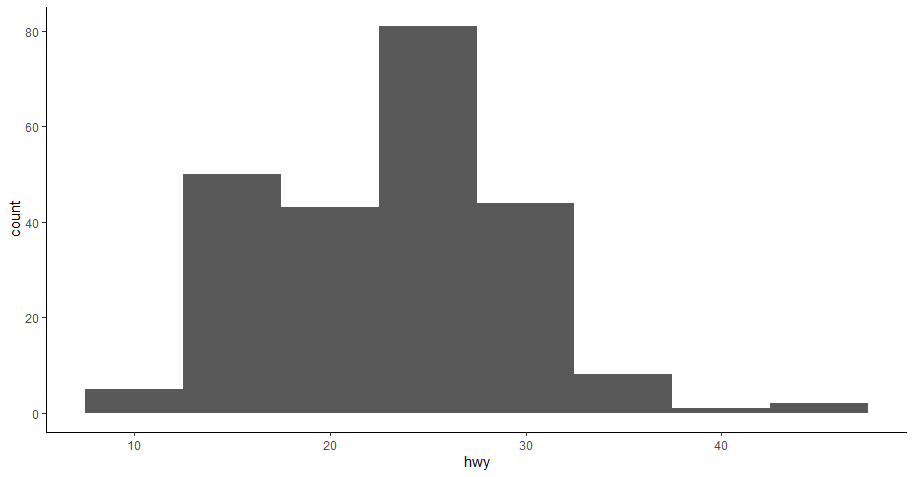The code above uses all four layers. Data and aesthetic are included in `ggplot(mpg, aes(hwy))`, then the `geom_histogram()` function added the geometrics of the histogram, and finally, `theme_classic()` is optional.

There are different types of geom functions available in `ggplot2` that can be used in different situations to create different plots. A geom is a geometric object that uses a plot to represent data, for example, a bar chart will use the bar geom, a line chart will use the line geom, and so on. This is reflected in the names of the geom functions as they are named accordingly, such as `geom_line()`, `geom_bar()`, etc.

In addition to primary layers, there are a few other useful features in `ggplot2` such as coordinate system, faceting, and statistical transformation, which we will explore in the remainder of this guide.

## Faceting

In faceting, you split a plot into multiple subplots using any categorical column or variable of the dataset. If you want to divide your plot using one variable, use the `facet_wrap()` function, and if you want to divide it using two variables, then use the `facet_grid()` function.

Let's apply both functions on the histogram you created earlier.

``````1# Saving histogram plot in a variable
2a <- ggplot(mpg, aes(hwy))+
3geom_histogram(binwidth = 5)+
4theme_classic()
5
6# Creating subplots using "cyl" column
7a + facet_wrap(~cyl)``````
html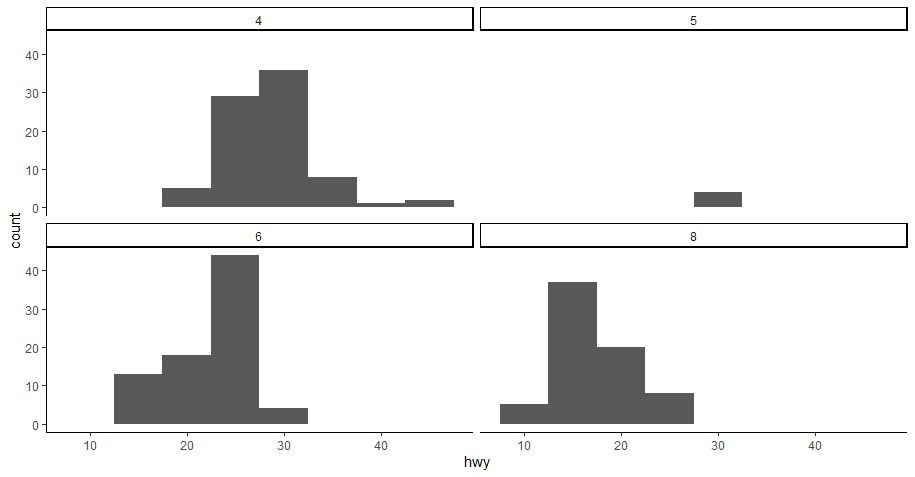As you can see, now we have four subplots, one for each unique value against the `cyl` column.

Now for the `facet_grid() function`. In this function, you will use two categorical columns from the dataset.

``````1# You will use 'a' variable storing the histogram again thus reducing code redundancy
2
3a + facet_grid(drv ~ cyl)``````
html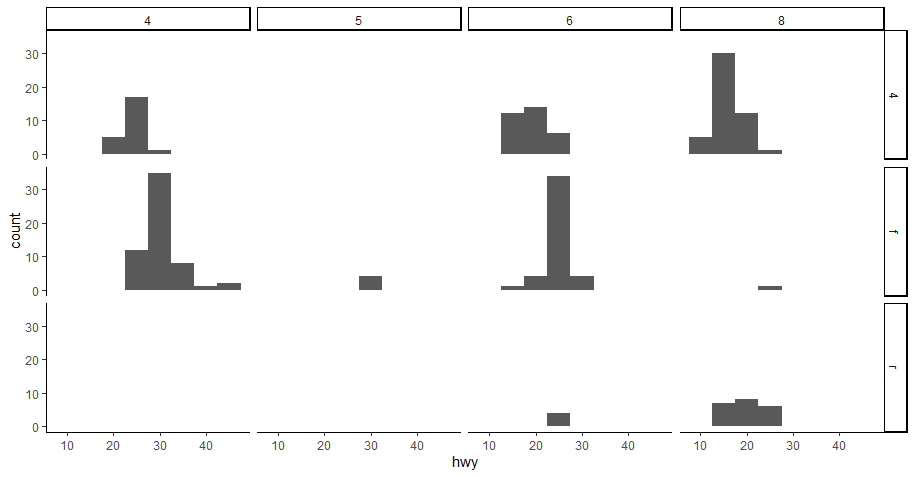## Coordinate System

The default coordinate system in `ggplot2` is a Cartesian coordinate system where the x and y positions are independent to locate a data point. There are different coordinate system functions in `ggplot2` that is used on different occasions. The most famous ones are `coord_flip()` and `coor_polar()`.

Let's look at a few examples to understand the use of `coord_flip()` and `coord_polar()`.

``````1# First create a bar chart, try to find yourself the reason for using  fill, show.legend, and width arguments
2
3bar <- ggplot(data = mpg) +
4     geom_bar(
5         aes(x = manufacturer, fill = manufacturer),
6         show.legend = FALSE,
7         width = 1  )
8
9bar``````
html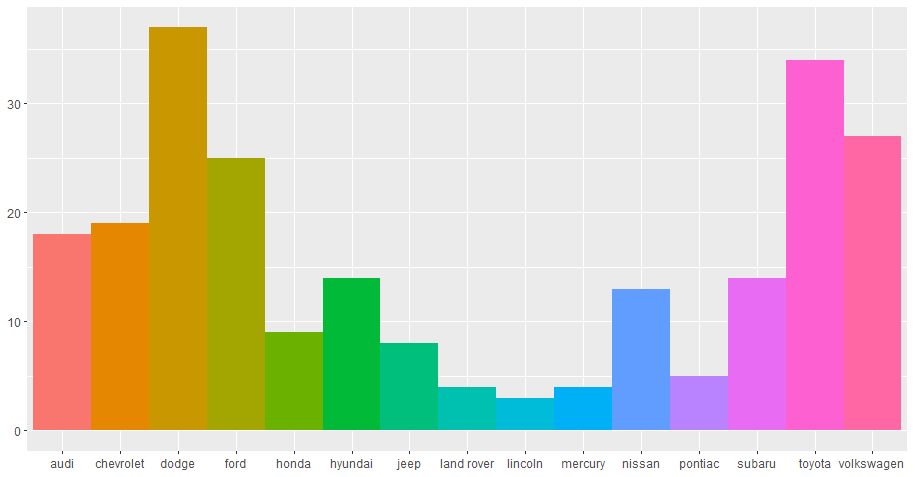``````1#lets use coord_flip()
2
3bar + coord_flip()``````
html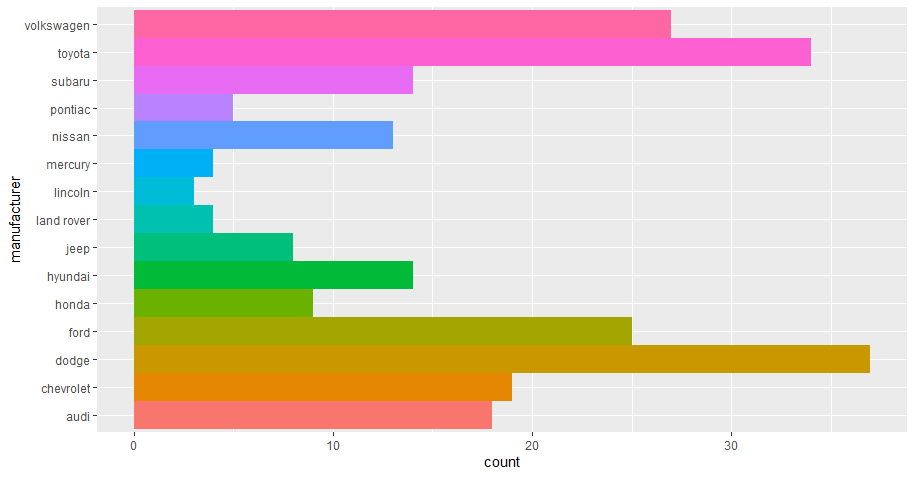As for `coord_polar()`,let's apply it to the same bar chart to use polar coordinates.

``1bar + coord_polar()``
html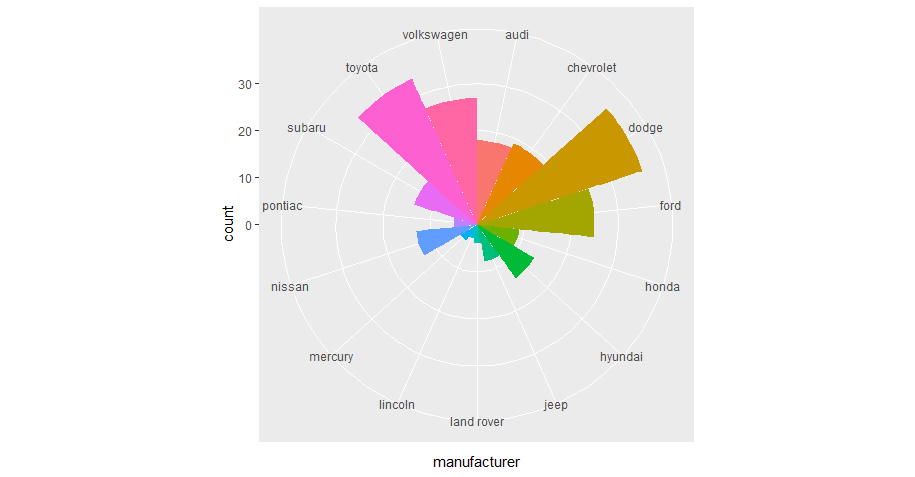## Statistical Transformation

If you look at the bar chart we created previously, you can see it shows additional information, including the count of records against each manufacturer, but the count is not available in the dataset. Some graphs show raw values, while others calculate new values and add them to the plot. The algorithm used to calculate new values for a graph is called a statistical transformation, or stat.

A bar graph can be created using the `stat_count()` function instead of `geom_bar()`. Every geom function has a default stat that can be overridden. See the example below.

``````1# Creating a dataset
2library(dplyr)
3a <- mpg%>%
4   group_by(manufacturer)%>%
5   summarise(Count = n())
6
7# Resetting the stat of geom_bar() from count to identity
8
9ggplot(data = a)+
10     geom_bar(mapping = aes(x = manufacturer, y = Count), stat = "identity")``````
html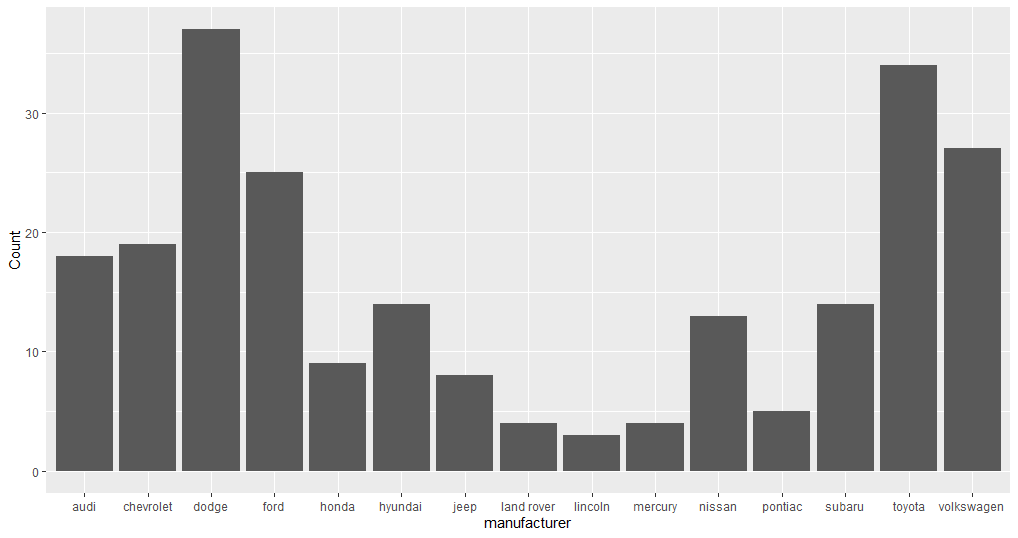## Applying All Layers

In the previous sections, you learned a foundation of creating a graph using `ggplot2` along with facets, coordinate systems, and statistical transformation. Now let's apply all of these using the template below.

``````1ggplot(data = <DATA>) +
2  <GEOM_FUNCTION>(
3     mapping = aes(<MAPPINGS>),
4     stat = <STAT>
5  ) +
6  <COORDINATE_FUNCTION> +
7  <FACET_FUNCTION>
8
9  ``````
html

Keep `stat = "count"`, which is a default, and use the mpg dataset only.

``````1ggplot(data = mpg)+
2 geom_bar(mapping = aes(x = manufacturer, fill = manufacturer),
3   stats = "count", show.legend = FALSE,
4         width = 1  )+
5   coord_flip()+
6   facet_wrap(~year)``````
html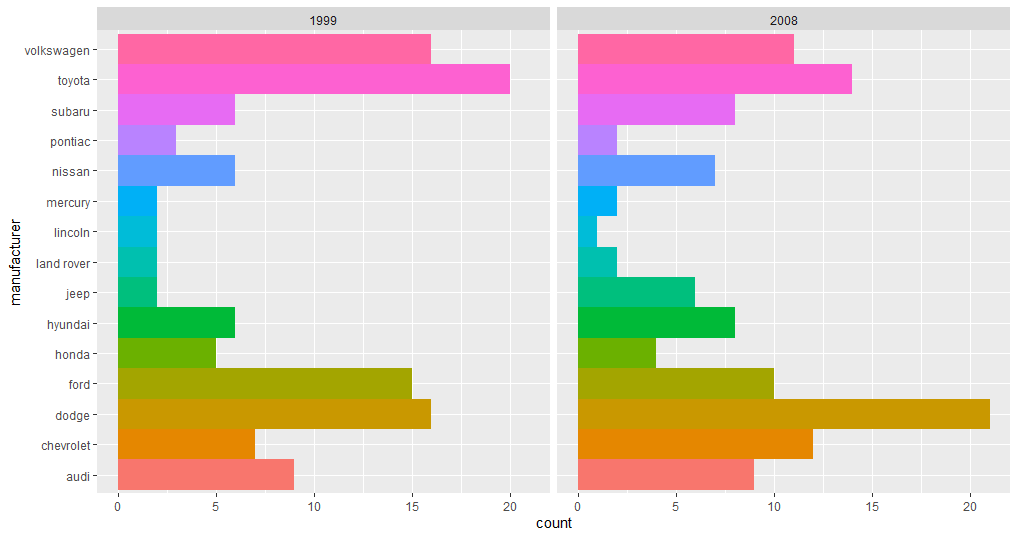## Conclusion

This guide explains the basics of creating a graph using `ggplot2` in R. You can also use `ggplot2` for your own data visualization requirements or in any data analysis project. There is a lot of flexibility when you are creating graphs with `ggplot2`. Each function contains a set of arguments that is available to tweak the graph accordingly. As a part of open source development, new features are added continually. This guide gives you a push to explore more in `ggplot2`.